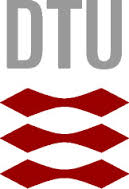#### Geometry Student Projects

Below are some examples of fagprojekter, Bachelor projects and Masters projects students could do with members of our group. The precise formulation of the project must be made with the help of a supervisor at the time of signing up for the project. Most projects can be adapted to different levels. We have tried to suggest an appropriate level for some of the projects. Students can also construct their own projects in consultation with group members. See also our Geometry Research page for information about our research.

#### Fagprojekt or Bachelor Project

• The shape of a Möbius strip: Take a strip of paper, twist it 180 degrees and attach the ends, forming a Möbius strip. The geometric shape minimizes the bending energy. Nobody knows the exact shape, but it can be approximated with the help of differential geometry and optimization.

• Foam geometry for exploring local and global consequences of the Plateau rules
A foam is organized according to three (Plateau) rules:The mean curvature of the surface between any two chambers of a soap film is everywhere constant and is proportional to the pressure difference between the two chambers. The soap film surfaces always meet in threes along an edge called a Plateau border, and they do so at an angle of arccos(-1/2). These Plateau borders meet in fours at a vertex, and they do so at an angle of arccos(-1/3) (the tetrahedral angle).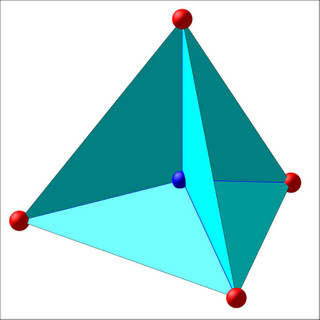The challenge is to understand these rules as local construction principles and then try to build a foam with prescribed properties or to extract general global geometric properties of any foam from the rules.

• The sound of a drum: Solve Helmholtz equation on a non-trivial domain in ${\mathbb R}^2$.

• MapleTA for computer assisted adaptive learning of differential geometry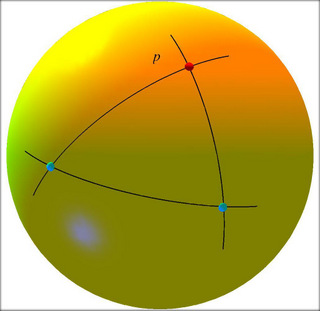This project is concerned with the study of the basics of computer assisted adaptive learning in mathematics together with the corresponding implementation into MapleTA. In particular, the learning of classical differential geometry of curves and surfaces will work as a first concrete target -- using visualization and basic knowledge of linear algebra as guiding assets towards e.g. Euler's theorem and/or the Gauss-Bonnet theorem.• Geometry of soap films Soap films are modelled by minimal surfaces. Minimal surfaces have a very nice representation by complex analytic functions, which gives one a powerful tool for studying this geometric problem. This is a good example of the link between abstract mathematics and concrete physical problems.

• Minimal surfaces: See the above description under Geometry of soap films". This project can be taken to a deeper level to make a Bachelor project.

#### Masters Project or Bachelor Project

• Metric graphs geometry for analyzing minimal and quantum webs on surfacesMinimal metric graphs are assumed to mimic a substantial amount of geometric and topological information of the surface (or manifold) in which they are minimal (with equipartition of directions at vertices and with geodesic edge segments). In this project we will study the extension of previously found results from the plane into the much more interesting domain of surfaces.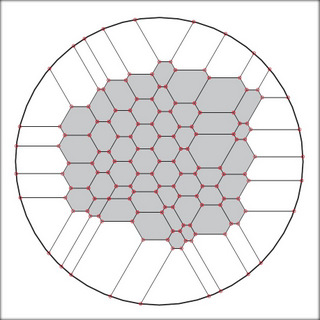• Experimental Geometry Using methods recently developed in integrable systems, we can compute many examples for various important surface classes, such as surfaces with constant Gaussian or mean curvature, and minimal surfaces. The global properties of these surfaces are not well understood, and numerical experiments give one the opportunity to explore the solution space and make conjectures.

• Lorentzian and semi-Riemannian geometry for working in General RelativityThe geometric framework of General Relativity is a rich and much needed tool for the proper understanding of relativistic phenomena such as gravitational lenses, black holes, big bangs, big crunches, and gravitational waves. In this project we will study Lorentzian and semi-Riemannian geometry to set up the fundamentals of this framework and look into such applications.

#### Open Category Projects

The projects below could be adapted to different levels, depending on how far you take them.
• Classical differential geometry for 3D printing of solid objects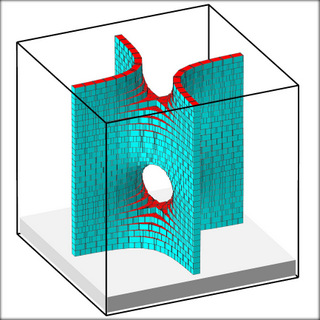In this project we study how to prepare a given surface for 3D printing via the setup of suitable triangulations in an *.stl file for printing with a powder printer. In particular curvature issues, orientation, and self-intersections, as well as measures of roughness of the final product will be considered.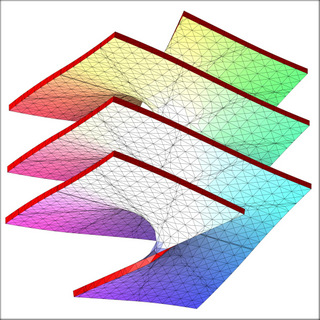• Geometry in Architecture: Various projects related to applicationis of differential geometry in architecture and design can be constructed, that relate well to some of the current research of our group members. Examples are: designing with ruled surfaces, designing with elastic curves, and developable surfaces. All of these are important classes of surfaces because of the constraints inherent in construction methods.

• Cartan ribbon geometry for sculpting and approximating doubly curved surfaces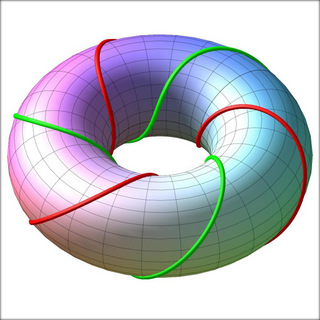In this project we cover and approximate (doubly curved) surfaces by perfectly flat strips, which are determined by pre-chosen steering curves on the given surfaces.• The sound of a non planar drum: Solve Helmholtz equation on a surface in ${\mathbb R}^3$, where the Laplace operator is replaced by the Laplace-Beltrami operator.

• The sound of a minimal surface: Given a closed curve in space, and a minimal surface with the given curve as the boundary, solve the Helmholtz equation.

• Lagrange-, Minkowski-, and Finsler-geometry for wildfire spread modelling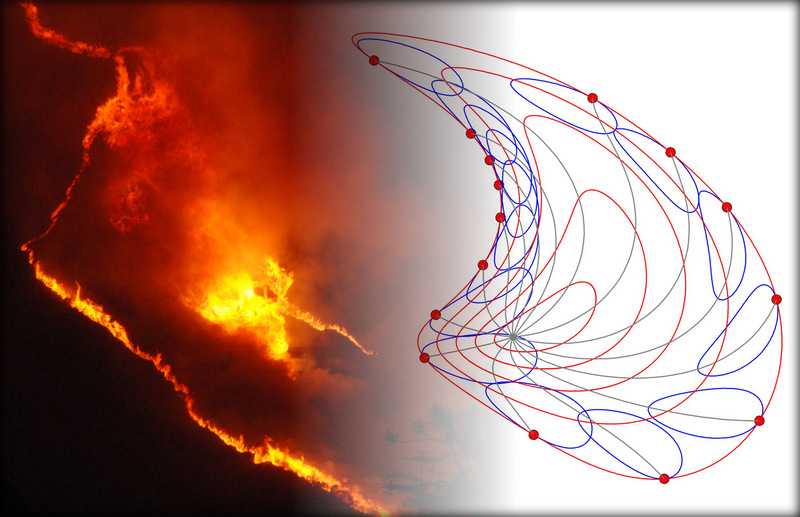Wildfire frontals are modelled by curves that foliate the domain through which the fire is burning. The spread mechanism is highly dependent on fuel properties, slope, and wind. In this project we use geometric analysis to encode these conditions into a system of geodesic differential equations whose solutions will mold the wildfire spread.

• Parametrisation in Isogemoetric Analysis: Perform a thorough testing of various parameterization methods proposed in: [ J. Gravesen, A. Evgrafov, Nguyen D.M., and P. Nrtoft, Planar Parametrization in Isogeometric Analysis and Shape Optimization", in Mathematical Methods for Curves and Surfaces, (Floater, M., Lyche, T., Mazure, M.-L., Mrken, K., Schumaker, L.L. (Eds.)), pp. 198{212, Springer, 2014. doi:10.1007/978-3-642-54382-1 ]

• Design of a harmonic gong: In [Nguyen D.M., A. Evgrafov, A.R. Gersborg, and J. Gravesen, Isogeometric shape optimization of vibrating membranes", Computer Methods in Applied Mechanics and Engineering 200, pp. 1343{1353, 2011, doi:DOI:10.1016/j.cma.2010.12.01 ] the shape of a "harmonic drum" is designed. What shape has a "harmonic gong"? It corresponds to replacing Dirichlet boundary condition with Neumann boundary condition.

• Heat conduction: Consider an annulus with different temperature on the inside and the outside. What shape has it if the gradient of the temperature is minimal?

• Dynamic waves: Consider a coast line and assume the water is at rest. Now let a storm start and end. How do the waves evolve? To simplify it a little assume that there is no variation along the coast so we only have two spatial dimensions.